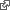#Positive and Negative Feedback

We construct a minimal dynamic system with both positive and negative feedback. We'll have two state variables, x and y, and render the phase trajectory as a dot.

digraph { rankdir=LR layout=neato node [shape=circle style=filled fillcolor=bisque] x -> y [label="+"] y -> x [label="-"] }

let x = 100 let y = 100 let g = 0.02 setInterval(()=>{ y += x * g x -= y * g },15)

We can interpret x*g and y*g as velocity that accumulates in the positions x and y. The velocity of x is proportional to the position y and vice versa.

http://ward.dojo.fed.wiki/assets/pages/positive-and-negative-feedback/index.html HEIGHT 400

We could derive velocity from acceleration caused by magnetic attraction. If everything were balanced just right then we would get the same motion. If anything were off then we get elliptical motion or worse, if we add a few more magnets we get chaos.

http://ward.dojo.fed.wiki/assets/pages/positive-and-negative-feedback/chaos.html HEIGHT 400

The inverse square attraction gets higher as the chance approach gets closer and that "slingshots" the velocity high enough for our dot to move out of view.

This is due to sampling error in my numerical integration of position and velocity from rapidly changing acceleration.

pages/positive-and-negative-feedback

See Magnetic Ribbon for a version dating back to 1996.

A pole-zero plot shows the location in the complex plane of the poles and zeros of the transfer function of a dynamic system, such as a controller, compensator, sensor, equalizer, filter, or communications channel. wikipedamitThis looks even better in 3d. Crazy. Maybe with some refactoring I can get a bunch of dots flying around.

http://ward.dojo.fed.wiki/assets/pages/positive-and-negative-feedback/crazy.html HEIGHT 400

We try a hierarchy of orbits around the sun, earth, moon and lunar orbiter trailing a long string. docdochttp://ward.dojo.fed.wiki/assets/pages/positive-and-negative-feedback/orbit.html HEIGHT 400

Note the string is in world coordinates with the sun but lead by orbiter coordinates transformed to world. docgraph { rankdir=LR scene -- sun -- earth -- moon -- orbiter scene -- string }